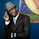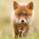1271 görüntülenme
1271
EXPERIMENTAL:
Counts the number of impulses with the same direction within a larger trend.
study(title='[RS]Convergence Divergence Impulse Counter V0', shorttitle='CDIC', overlay=false)
length01 = input(20)
length02 = input(100)
src = input(close)
ma01 = ema(src, length01)
ma02 = ema(src, length02)

cd01 = src - ma01
cd02 = ma01 - ma02

imp = src > ma01 and ma01 > ma02 ? cd01 : src < ma01 and ma01 < ma02 ? cd01 : 0
count = na(count) ? 0 :
change(crossover(cd02, 0)) > 0 ? 1 :
change(crossunder(cd02, 0)) > 0 ? -1 :
count > 0 and change(crossover(cd01, 0)) > 0 ? count + 1 :
count < 0 and change(crossunder(cd01, 0)) > 0 ? count - 1 :
count

//plot(series=imp, color=black, style=columns)
//plot(series=cross(cd02, 0) ? 0 : na, color=color(aqua, 0), style=circles, linewidth=4)
plot(series=count, color=black, style=columns)
hline(0)Can you, please, make an other like this to counts the number of bars between 2 min( short and long series) and 2 max and just show the number of bars ? Thank yuo
Cevap GönderAre there any examples of this elsewhere; or books to read to gain the knowledge to understand how to apply this tool to trading impulses?
Cevap GönderRocketman
not that im aware, from observation the higher the count the higher the risk of the trade going flat/turning
Cevap Gönder